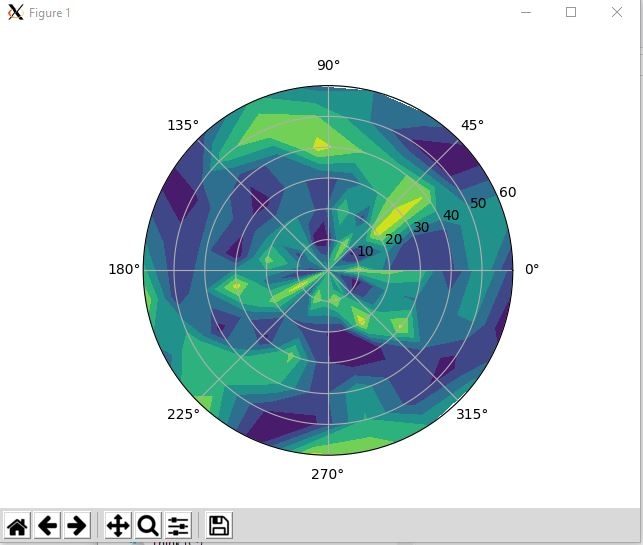# Python 画极坐标图

--------------------------------------------------

import numpy as np
import matplotlib.pyplot as plt

#-- Generate Data -----------------------------------------
# Using linspace so that the endpoint of 360 is included...
zeniths = np.arange(0, 70, 10)

r, theta = np.meshgrid(zeniths, azimuths)
values = np.random.random((azimuths.size, zeniths.size))

#-- Plot... ------------------------------------------------
fig, ax = plt.subplots(subplot_kw=dict(projection='polar'))
ax.contourf(theta, r, values)

plt.show()fig, ax = subplots(subplot_kw=dict(projection='polar'))
cax = ax.contourf(theta, r, values, nlevels)

Radius　　　Theta　　　　Value
10　　　　　　0　　　　　  0.7
10　　　　　 90　　　　　 0.45
10　　　　  180 　　     0.9
10    　　　270   　　  0.23
20　　　　   0    　　   0.5
20    　　　90    　　  0.13
20    　　 180    　　 0.52
20        270 　　    0.98

这些行中的每一行都定义一个点 - 例如，第一行定义半径为10的点，角度为0度，值为0.7。我永远无法理解为什么轮廓功能不只是采用这三个列表并绘制一个等高线图。事实上，我已经编写了一个函数来完成这个，我将在下面描述，但首先让我解释一下这些值是如何转换为二维数组的。

       0      90     180    270
10    0.7    0.45    0.9    0.23
20    0.5    0.13    0.52    0.98

这正是我们需要为contourf函数赋予的那种二维数组。这并不难理解——但为什么地球上的半径和角度数组也必须是二维的。好吧，基本上我们只需要两个像上面那样的数组，但是在对应的单元格中有相应的半径和角度，而不是值。所以，对于角度，我们有：

      0    90    180    270
10    0    90    180    270
20    0    90    180    270

      0    90    180   270
10    10   10    10    10
20    0    20    20    20

>>> radii = np.arange(0, 60, 10)
[ 0 10 20 30 40 50]
>>> angles = np.arange(0, 360, 90)
>>> print(angles)
[  0  90 180 270]
[array([[  0,  90, 180, 270],
[  0,  90, 180, 270],
[  0,  90, 180, 270],
[  0,  90, 180, 270],
[  0,  90, 180, 270],
[  0,  90, 180, 270]]), array([[ 0,  0,  0,  0],
[10, 10, 10, 10],
[20, 20, 20, 20],
[30, 30, 30, 30],
[40, 40, 40, 40],
[50, 50, 50, 50]])]ax.set_theta_zero_location("N")
ax.set_theta_direction(-1)

 1 import numpy as np
2 from matplotlib.pyplot import *
3
4 def plot_polar_contour(values, azimuths, zeniths):
5     """Plot a polar contour plot, with 0 degrees at the North.
6
7     Arguments:
8
9      * values -- A list (or other iterable - eg. a NumPy array) of the values to plot on the
10      contour plot (the z values)
11      * azimuths -- A list of azimuths (in degrees)
12      * zeniths -- A list of zeniths (that is, radii)
13
14     The shapes of these lists are important, and are designed for a particular
15     use case (but should be more generally useful). The values list should be len(azimuths) * len(zeniths)
16     long with data for the first azimuth for all the zeniths, then the second azimuth for all the zeniths etc.
17
18     This is designed to work nicely with data that is produced using a loop as follows:
19
20     values = []
21     for azimuth in azimuths:
22       for zenith in zeniths:
23         # Do something and get a result
24         values.append(result)
25
26     After that code the azimuths, zeniths and values lists will be ready to be passed into this function.
27
28     """
30     zeniths = np.array(zeniths)
31
32     values = np.array(values)
33     values = values.reshape(len(azimuths), len(zeniths))
34
35     r, theta = np.meshgrid(zeniths, np.radians(azimuths))
36     fig, ax = subplots(subplot_kw=dict(projection='polar'))
37     ax.set_theta_zero_location("N")
38     ax.set_theta_direction(-1)
39     autumn()
40     cax = ax.contourf(theta, r, values, 30)
41     autumn()
42     cb = fig.colorbar(cax)
43     cb.set_label("Pixel reflectance")
44
45     return fig, ax, cax

posted @ 2019-05-04 20:05  chinagod  阅读(12828)  评论(0编辑  收藏  举报